# Testing and Correcting the Linearity of Pellistor Sensors

The response of a pellistor sensor to flammable gases is linear at levels of around 60% of the lower explosive limit (LEL). The deviation of this linearity at gas levels close to the LEL is indicated as reduced signal per %LEL. Therefore, sensors are regularly subjected to performance tests for evaluating their linearity over the operating range.

Due to this non-linearity at higher gas levels, instruments that can be used up to the LEL may experience issues when subjected to performance tests. It is necessary to consider the following effects for linearity corrections to the response of pellistor sensors at high gas levels.

## Diffusion Considerations

The pellistors are normally placed in a flameproof enclosure that also contains a sintered metal flame arrester, which is the first diffusion barrier for the target gas. This gas has to subsequently pass through a second barrier at the entrance to the sensor before reaching the proximity of the sensor beads. Once the gas reaches close to the sensor beads, a depletion layer that develops around the detector surface due to the diffusion of combustion products away from the detector acts as a third barrier.

The rate at which the fuel reaches the catalytic surface and the rate at which the gas diffuses to the catalyst’s surface determine the pellistor response. This rate determining step, rd is expressed in the following Equation 1.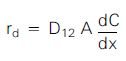(1)

Where, D12 is the binary diffusion coefficient, A is the cross-sectional area through which the gas diffuses, C is the bulk gas concentration, and x is the distance from the diffusion barrier. When there is no change in the sintered metal and the sensor can, there will be an increase in the thickness of the depletion layer with increasing gas concentration at a fixed ambient pressure.

This results in a non-linear increase in the diffusion time of fuel to the catalyst surface relating to the concentration of fuel. The fuel concentration as a function of distance diffused by the gas through the depletion layer is expressed in Equation 2: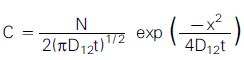(2)

Where, C is the concentration at a distance ‘x’ from the diffusion barrier after a time ‘t’ from an initial number of molecules ‘N’ at time ‘zero’. This decreases the diffusive flux of molecules within the vicinity of the catalyst surface with increasing thickness of the depletion layer. When there are increased effects of external diffusion controlling elements, there will be a reduced effect of diffusion through the depletion layer. Consequently, the signal linearity enhances at high gas levels.

The speed at which the fuel reacts on a pellistor is diffusion controlled under standard operating conditions. Thus, the reaction at the surface of the catalyst is not usually rate determining, following a heterogeneous mechanism based on the reactants’ surface coverage. The rate of reaction on the catalyst surface becomes the rate determining step at low operating temperatures. The steps involved in surface catalysis are as follows:

• Diffusion of reactants to the catalyst surface
• Adsorption of reactants at the catalyst surface
• Chemical reaction on the catalyst surface
• Desorption of products from the catalyst surface
• Diffusion of products from the catalyst surface

The reaction of adsorbed molecules turns out to be the rate determining step for treating the kinetics of these surface reactions. The rate of reaction per unit surface area changes in relation to the part of a surface covered as expressed by a Langmuir isotherm. The associated reaction rate is described in Equation 3 as follows: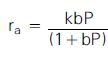(3)

Where k is the rate constant; b is the adsorption coefficient; P is the partial pressure of fuel. With increasing fuel concentration, the non-linearity is introduced by the term (1 + bP).

## Constant Voltage Responses

The out-of-balance voltage acquired from a sensor bridge configuration supplied with a constant voltage in terms of the resistances of the detector and compensator is explained in Equation 4: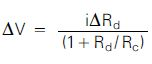(4)

Where, ÄV is the voltage signal, i is the current through the detector, ÄRd is the change in the detector resistance; Rd / RC is the compensator resistance.

## Non-Linearity Estimation

The non-linearity is described in the following Equation 5: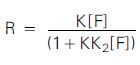(5)

Where R is the overall response, K is the normal linear slope of response against fuel concentration (F), and K2 is the non-linear slope contribution. The following equation expresses the reciprocal form of Equation 5: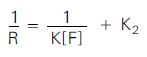(6)

In the above equations, a value is introduced for K2 for non-linear correction. When the K2 value is zero, there will be a linear sensor response and also minimal impact of the non-linear contribution at low fuel concentrations. The value of the K2 value can possibly be estimated by quantifying the response at two calibration points of the fuel.

For example, while exposing VQ542R sensors to 50% LEL methane and 100% LEL, the response ratio differs between 1.75 and 1.95, with a linear relationship leading to a factor of 2.0. From these values, the mean signal ratio is around 1.85 (R100 = 1.85 x R50) and therefore: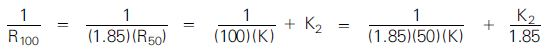(7)

Equation 7 results in the following expression: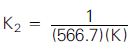(8)

After including the relationship between constants in Equation 8, Equation 6 is rearranged as follows: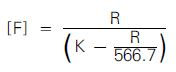(9)

Where, F is the fuel concentration to be read; R is the sensor response signal; and K is the slope for signal calibration at a single gas concentration.

The effect of using Equation 9 for the VQ542R miniature sensing heads is summarized in the following table:

Gas Conc. (%LEL) R (1.75) (mV) Linear (1.75) (%LEL) [F] calc. (%LEL) R (1.85) (mV) Linear (1.85) (%LEL) [F] calc. (%LEL) R (1.95) (mV) Linear (1.95) (%LEL) [F] calc. (%LEL)
0 0 0 0 0 0 0 0 0 0
10 17 11 11 16 11 10 15 10 9
20 33 22 21 32 21 20 30 20 19
30 48 32 31 47 31 30 45 30 29
40 62 41 41 61 41 40 60 40 39
50 75 50 50 75 50 50 75 50 50
60 87 58 59 89 59 60 89 59 60
70 99 66 68 102 68 70 103 69 71
80 110 73 77 114 76 80 117 78 82
90 121 81 85 127 85 90 132 88 94
100 131 87 94 139 93 100 146 97 106

## Conclusion

Based on the range of response ratios Ri00/R50 from 1.75 to 1.95, Equation 9 can be applied to different e2v technologies sensors. However, the effect of the sensor housing on the linearity of the sensor response needs a different K2 value as a result of its contribution to smaller response ratios. In the above example of VQ542R sensors, the effects of sensor housing have been included.This information has been sourced, reviewed and adapted from materials provided by SGX Sensortech (IS) Ltd.

## Citations

• APA

SGX EUROPE SP. Z O.O.. (2020, March 13). Testing and Correcting the Linearity of Pellistor Sensors. AZoSensors. Retrieved on August 10, 2020 from https://www.azosensors.com/article.aspx?ArticleID=540.

• MLA

SGX EUROPE SP. Z O.O.. "Testing and Correcting the Linearity of Pellistor Sensors". AZoSensors. 10 August 2020. <https://www.azosensors.com/article.aspx?ArticleID=540>.

• Chicago

SGX EUROPE SP. Z O.O.. "Testing and Correcting the Linearity of Pellistor Sensors". AZoSensors. https://www.azosensors.com/article.aspx?ArticleID=540. (accessed August 10, 2020).

• Harvard

SGX EUROPE SP. Z O.O.. 2020. Testing and Correcting the Linearity of Pellistor Sensors. AZoSensors, viewed 10 August 2020, https://www.azosensors.com/article.aspx?ArticleID=540.